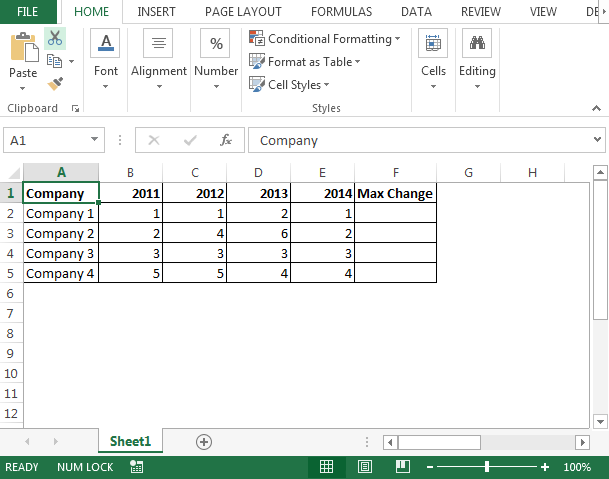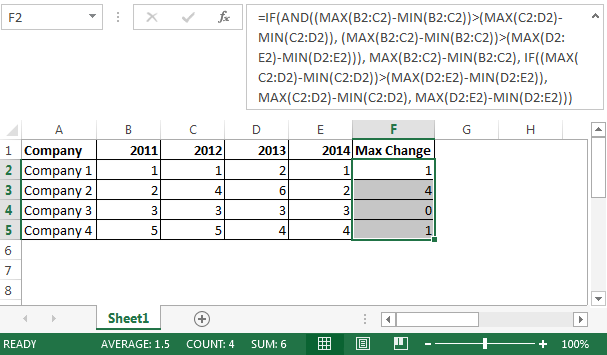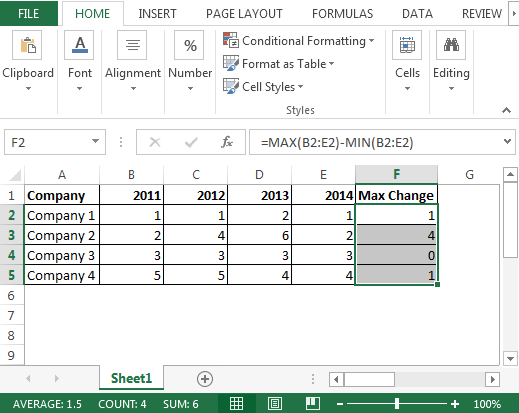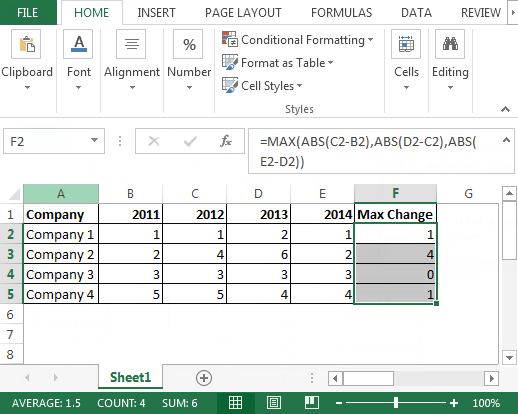Avoid Nested IF in Microsoft Excel

In case we are using nested IF formula & we all know nested IF takes a lot of time in calculating the result. In this article, we will learn how we can avoid using nested IF function.

Question: I have a task assigned by my manager to check / compare the max change in the last four years sales figures.

I tried using IF function along with MAX & MIN functions to get the result but I guess the formula I am using is a bit long & I want to avoid; so if there is any formula that can replace nested IF then it will be a great help for me.

Following is the snapshot of data we have; the numbers may mislead you but these all are in million• The formula is needed to check the max change between two years data
• The formula we are going to use is
• =IF(AND((MAX(B2:C2)-MIN(B2:C2))>(MAX(C2:D2)-MIN(C2:D2)), (MAX(B2:C2)-MIN(B2:C2))>(MAX(D2:E2)-MIN(D2:E2))), MAX(B2:C2)-MIN(B2:C2), IF((MAX(C2:D2)-MIN(C2:D2))>(MAX(D2:E2)-MIN(D2:E2)), MAX(C2:D2)-MIN(C2:D2), MAX(D2:E2)-MIN(D2:E2)))• There are two formulas we can use & both of them involve MAX, MIN & ABS functions
• In cell F2, the formula is
• =MAX(B2:E2)-MIN(B2:E2)• The second formula is
• =MAX(ABS(C2-B2),ABS(D2-C2),ABS(E2-D2))Conclusion: In this way, we can avoid using nested IF function & we can opt for other functions.We would love to hear from you, do let us know how we can improve, complement or innovate our work and make it better for you. Write us at info@exceltip.com

1.Hi,

Honestly, didn't crossed my mind the nested if solution.
On the other hand it seems to me obviously the max-min solution.

Good job. Keep on.

Vândalo

Terms and Conditions of use

The applications/code on this site are distributed as is and without warranties or liability. In no event shall the owner of the copyrights, or the authors of the applications/code be liable for any loss of profit, any problems or any damage resulting from the use or evaluation of the applications/code.